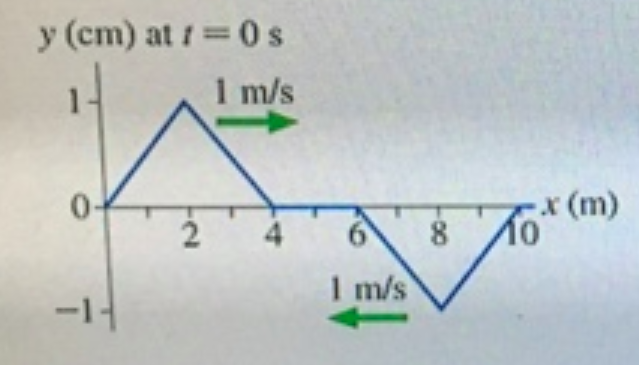# Problem: Figure 1 is a snapshot graph at t=0s of two waves on a string approaching each other at 1 m/sList the values of the displacement of the string at x = 5.0 cm at 1 s intervals from t = 0 s to t = 6 s. Express your answer using two significant figures separated by commasy0 • y1 • y2 • y3 • y4 • y5 • y6 =

###### FREE Expert Solution

We're told that the speed of each wave is 1.0 m/s.

This means that the waves move 1.0m in each second.

81% (319 ratings)###### Problem Details

Figure 1 is a snapshot graph at t=0s of two waves on a string approaching each other at 1 m/sList the values of the displacement of the string at x = 5.0 cm at 1 s intervals from t = 0 s to t = 6 s. Express your answer using two significant figures separated by commas

y• y1 • y2 y3 • y4 • y5 • y6 =